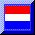# Theory for Halton Arp

## Mathematics: Han de Bruijn

Key reference is the monograph by Barry Setterfield: Cosmology and the Zero Point Energy (Natural Philosophy Alliance Monograph series, No.1, 2013, ISBN 978-1-304-19508-1).
One of the statements in this monograph is that the rest mass of an electron has been increasing with time, as shown in e.g. Figure 4 on page 17 and figure 8 on page 395 (linked here):My Theory for Halton Arp rests on a wild hypothesis : the (rest) mass of atomic particles is increasing with (proper) time.
What is the truth value of such a highly speculative theory?
Time to give a little lesson in what the scientific method is about, especially meant for those who think that what they think must be true, because they wishfully think so.
Let $H =$ Hypothesis, $C =$ Conclusion and let "if $H$ then $C$" be our theory, then we have the following possibilities$^{(*)}$:

 H C if H then C true true true true false false false true true false false true

This means that our theory can only be false if the Hypothesis is true while any of the Conclusions is false. So it's still true if the Hypothesis is false. Which is pretty good :-)

### SamenvattingBasic HypothesisIntrinsic RedshiftLength ContractionGiant CreaturesTime DilationNarlikar's LawMilne's FormulaVan Flandern'sCreation TimePendulum testC-decay TheoriesC-decay data fitBarry's pictureUpside DownGalaxy projectHubble Trouble

(*) Material conditional, Conditional Statements: if p then q, Propositional Logic and Redundancy.